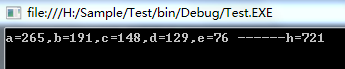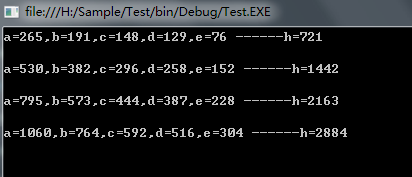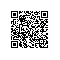# 经典算法题每日演练——第二题 五家共井

古代数学巨著《九章算数》中有这么一道题叫“五家共井，甲二绠（汲水用的井绳）不足，如（接上）乙一绠；乙三绠不足，如丙一绠；

我们设井深为h，各家分别为a,b,c,d,e，则可以列出如下方程组：

2a+b=h   ①

3b+c=h   ②

4c+d=h   ③

5d+e=h   ④

6e+a=h   ⑤

a=b+c/2

b=c+d/3

c=d+e/4

d=e+a/5

 1 namespace Test
2 {
3     class Program
4     {
5         static void Main(string[] args)
6         {
7             int a, b, c, d, e, h;
8
9             a = b = c = d = e = h = 0;
10
11             bool flag = true;
12
13             while (flag)
14             {
15                 //4的倍数
16                 e += 4;
17
18                 a = 0;
19
20                 while (flag)
21                 {
22                     //5的倍数
23                     a += 5;
24
25                     d = e + a / 5;
26
27                     c = d + e / 4;
28
29                     if (c % 2 != 0)
30                         continue;
31
32                     if (d % 3 != 0)
33                         continue;
34
35                     b = c + d / 3;
36
37                     if (b + c / 2 < a)
38                         break;
39
40                     if (b + c / 2 == a)
41                         flag = false;
42                 }
43             }
44
45             h = 2 * a + b;
46
47             Console.WriteLine("a={0},b={1},c={2},d={3},e={4} ------h={5}\n", a, b, c, d, e, h);
48
50         }
51     }
52 }b=h-2a   ⑥

c=h-3b   ⑦

d=h-4c   ⑧

e=h-5d   ⑨

a=h-6e   ⑩

c=(148/721)h

a=265k

b=191k

c=148k

d=129k

e=76k

x=721k

 1 namespace Test
2 {
3     class Program
4     {
5         static void Main(string[] args)
6         {
7             for (int k = 1; k < 5; k++)
8             {
9                 int h = 721 * k;
10
11                 int a = 265 * k;
12
13                 int b = 191 * k;
14
15                 int c = 148 * k;
16
17                 int d = 129 * k;
18
19                 int e = 76 * k;
20
21                 Console.WriteLine("a={0},b={1},c={2},d={3},e={4} ------h={5}\n", a, b, c, d, e, h);
22             }
23
25         }
26     }
27 }使用钉钉扫一扫加入圈子
+ 订阅